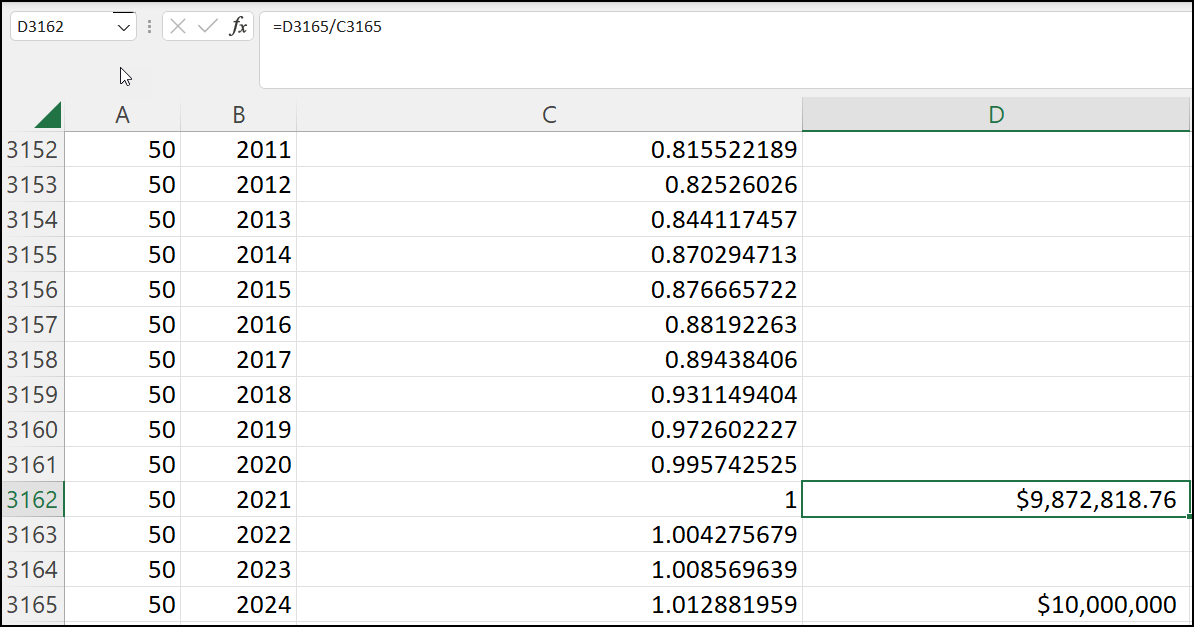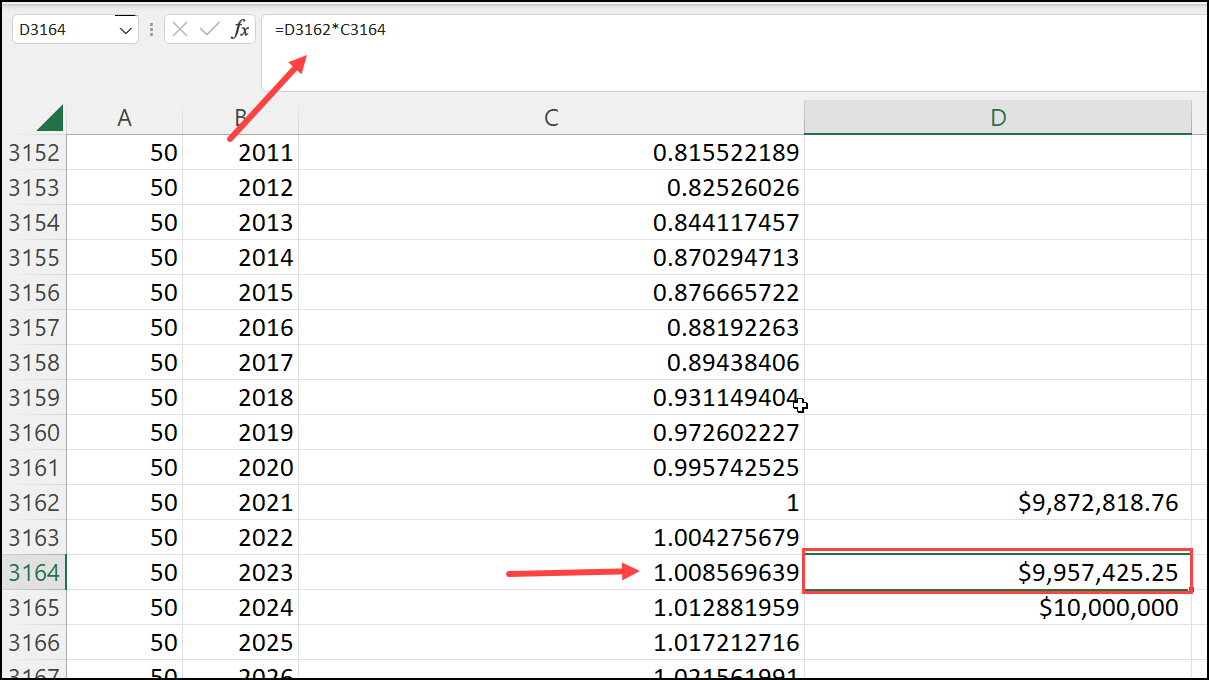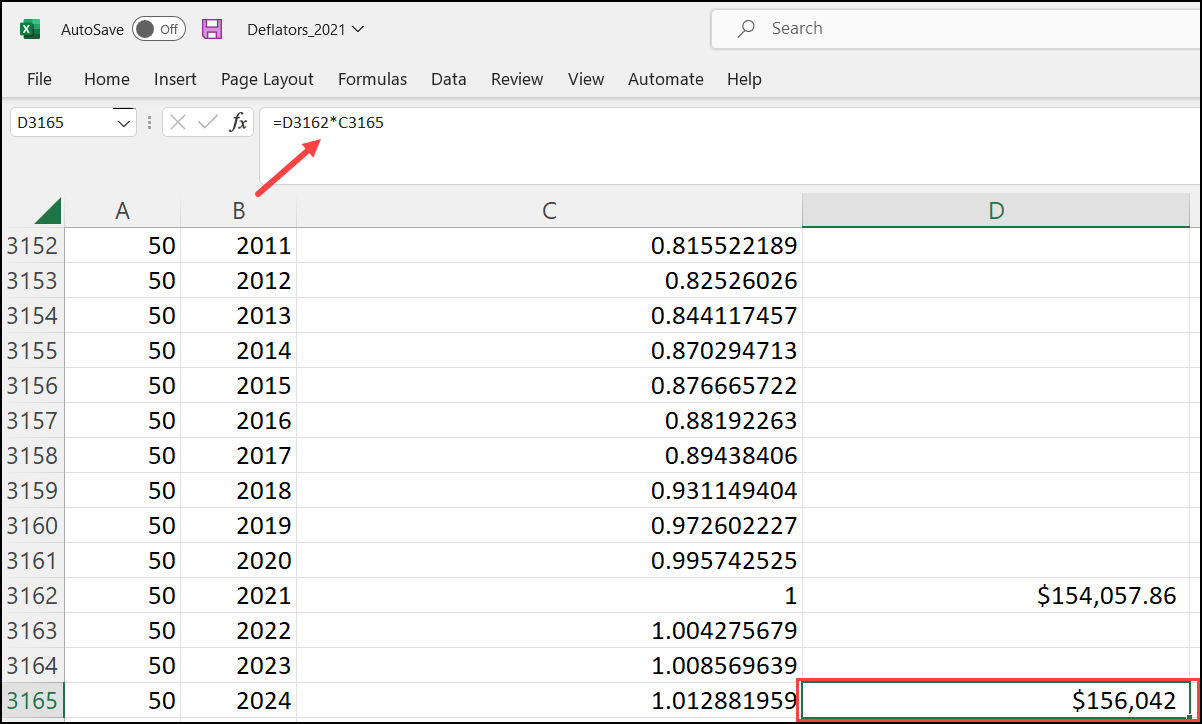1 comment

• Official commentHello!

So i'll walk you through a quick example to show how the deflators will be applied to your Event Value: say that we ran \$10M through Industry 50, in 2024 Dollar Year using the 2021 Data Year. First, IMPLAN will divide this \$10M by the Output deflator from 2021-2024, leaving us with a Direct Output of \$9,872,818,76 in 2021 values.So if you viewed the Results in 2021 Dollar Year, this is the Direct Output you would see. If for instance you viewed in 2023 Dollar Year, IMPLAN would then multiply this \$9.87M by the Deflator for 2023:In the case of a standard Industry Event in IMPLAN, Direct jobs are in fact calculated by dividing the Direct Output by the Output per worker, however, remember that the Output per worker value from the Region Details is in nominal values. So, before this calculation is performed, the nominal value is inflated/deflated to account for the Industry and Dollar year you choose.

Sticking with our example from above, say this was for the US totals (2021), where the Output per worker is \$154,057.86 for Industry 50. We would take this value and multiply it by the deflator from 2021 to 2024:We would then divide our \$10M in Industry 50 Output by this \$156,042 to get a Direct Employment of roughly 64.09. However, I would note that the Industry Impact Analysis Event does not follow this same logic for calculating Direct Employment.

Hope this helps!

Michael Nealy